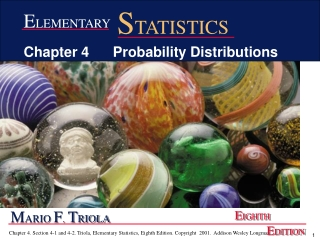# M ARIO F . T RIOLA - PowerPoint PPT PresentationDownload PresentationM ARIO F . T RIOLA

M ARIO F . T RIOLADownload Presentation## M ARIO F . T RIOLA

- - - - - - - - - - - - - - - - - - - - - - - - - - - E N D - - - - - - - - - - - - - - - - - - - - - - - - - - -
##### Presentation Transcript

1. STATISTICS ELEMENTARY Chapter 4 Probability Distributions MARIO F. TRIOLA EIGHTH EDITION

2. Chapter 4Probability Distributions 4-1 Overview 4-2 Random Variables 4-3 Binomial Probability Distributions 4-4 Mean, Variance, Standard Deviation for the Binomial Distribution

3. 4-1 Overview This chapter will deal with the construction of probability distributions by combining the methods of Chapter 2 with the those of Chapter 3. Probability Distributions will describe what will probably happen instead of what actually did happen.

4. Combining Descriptive Statistics Methods and Probabilities to Form a Theoretical Model of Behavior Figure 4-1

5. 4-2 Random Variables

6. Definitions • Random Variable a variable (typically represented by x) that has a single numerical value, determined by chance, for each outcome of a procedure • Probability Distribution a graph, table, or formula that gives the probability for each value of the random variable

7. Table 4-1 Probability DistributionNumber of Girls Among Fourteen Newborn Babies x P(x) 0 1 2 3 4 5 6 7 8 9 10 11 12 13 14 0.000 0.001 0.006 0.022 0.061 0.122 0.183 0.209 0.183 0.122 0.061 0.022 0.006 0.001 0.000

8. Probability Histogram Figure 4-3

9. Definitions • Discrete random variable has either a finite number of values or countable number of values, where ‘countable’ refers to the fact that there might be infinitely many values, but they result from a counting process. • Continuous random variable has infinitely many values, and those values can be associated with measurements on a continuous scale with no gaps or interruptions.

10. Requirements for Probability Distribution The sum of all the probabilities of the distribution equals 1. ΣP(x) = 1 where x assumes all possible values All probabilities of the distribution must fall between 0 and 1 inclusive. 0 P(x) 1 for every value of x

11. Mean, Variance and Standard Deviation of a Probability Distribution Mean µ = [x•P(x)] Variance 2= [(x - µ)2 • P(x)] Standard Deviation = 2

12. Roundoff Rule for µ, 2, and Round results by carrying one more decimal place than the number of decimal places used for the random variable x. If the values of xare integers, round µ, 2, and  to one decimal place.

13. Example A 4 point pop quiz was given and the scores ranged from 0 to 4, with the corresponding probabilities: 0.05, 0.2, 0.25, 0.3, 0.2 • Write the probability distribution in a table. • Verify whether a probability distribution is given. • Compute the mean, variance and standard deviation of the probability distribution.

14. Solution: Write the probability distribution in a table.

15. Solution: Verify whether a probability distribution is given • Yes it is a probability distribution because the sum of P(x) for all values of x is 1 and P(x) for all values of x is between 0 and 1, inclusive.

16. Solution: Compute the mean • Enter x values into L1, Enter P(x) into L2. • Compute the products L3 = L1* L2. • Find the sum(L3) which is the Mean: μ = 2.4

17. Solution: Compute the variance and standard deviation. • Having already found the mean of 2.4 • Compute L4 = (L1- 2.4)2* L2. • Find the sum(L4) which is the 2 = 1.34  1.3 • Find the  which is the square root of 2 = = 1.16  1.2 • Mean: μ = 2.4 Variance: σ2= 1.3 Std Dev σ = 1.2

18. Definition Expected Value The average(mean) value of outcomes, if the trials could continue indefinetly. E =  [x • P(x)]

19. The Daily Number allows you to place a bet that the three-digit number of your choice. It cost \$1 to place a bet in order to win \$500. What is the expected value of gain or loss?

20. E =  [x • P(x)] Event Win Lose x \$499 - \$1

21. E =  [x • P(x)] Event Win Lose x \$499 - \$1 P(x) 0.001 0.999

22. E =  [x • P(x)] Event Win Lose x \$499 - \$1 P(x) 0.001 0.999 x • P(x) 0.499 - 0.999

23. E =  [x • P(x)] Event Win Lose x \$499 - \$1 P(x) 0.001 0.999 x • P(x) 0.499 - 0.999 E = -\$.50

24. Expected Value • This means that in the long run, for each \$1 bet, we can expect to lose an average of \$.50. • In actuality a player either loses \$1 or wins \$499, there will never be a loss of \$.50. • If an expected value is \$0, that means that the game is fair, and favors no side of the bet.

25. Alternate Solution: Excluding the cost of playing. Event Win Lose x \$500 0 P(x) 0.001 0.999 x • P(x) 0.50 0 E = \$.50, Which means the average win is \$.50. If the game cost \$.50 to play E = 0 and it would be a “fair” game and not favor either side of the bet. If the game cost \$.75 to play E = -.25 and it would not be a “fair” game and would favor the house.